# Reduced cohomology

<]]>

<]]>

<]]>

<]]>

<]]>
• ## Properties

See Generalized cohomology for some notation.

A reduced cohomology theory is a sequence of functors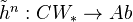together with natural transformations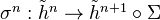, satisfying the following axioms:

• IV' (Exactness): For any inclusion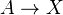in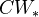, the sequence: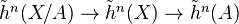is exact
• V' (Homotopy): Homotopic morphisms ininduce the same map on cohomology
• VI' (Suspension): For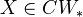, the map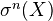is an isomorphism for all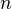.

We can also consider an additivity axiom, saying that "the cohomology of a wedge product is the product of the individual cohomology groups".

<]]>
• ## Standard theorems

The reduced cohomology theories are in bijection with the generalized cohomology theories under composition with the functor from CW pairs tosending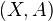to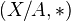.

If a map of pointed CW complexes induces an isomorphism on ordinary homology, then it also induces an isomorphism on any reduced cohomology theory. If the complexes involved are finite, the same holds for ordinary cohomology.

(Milnor exact sequence): For a theory satisfying additivity, we have, for any nested union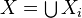, an exact sequence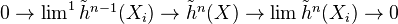Roughly, the following is true: A cohomology theory on finite CW complexes is determined by its Coefficient group, and the same is true for a theory on CW complexes, provided it satisfies additivity.

<]]>

<]]>

<]]>

<]]>

<]]>

<]]>

<]]>